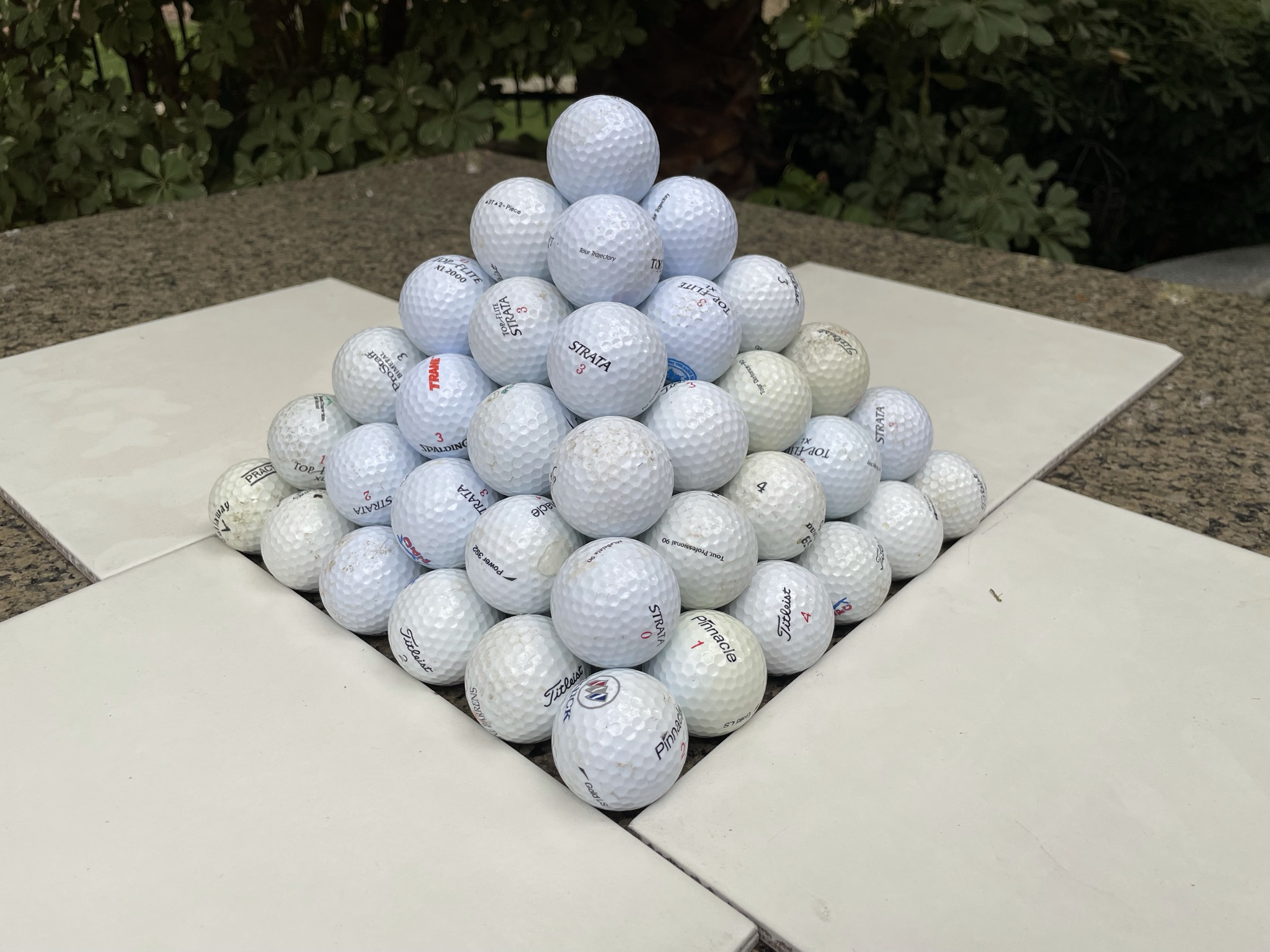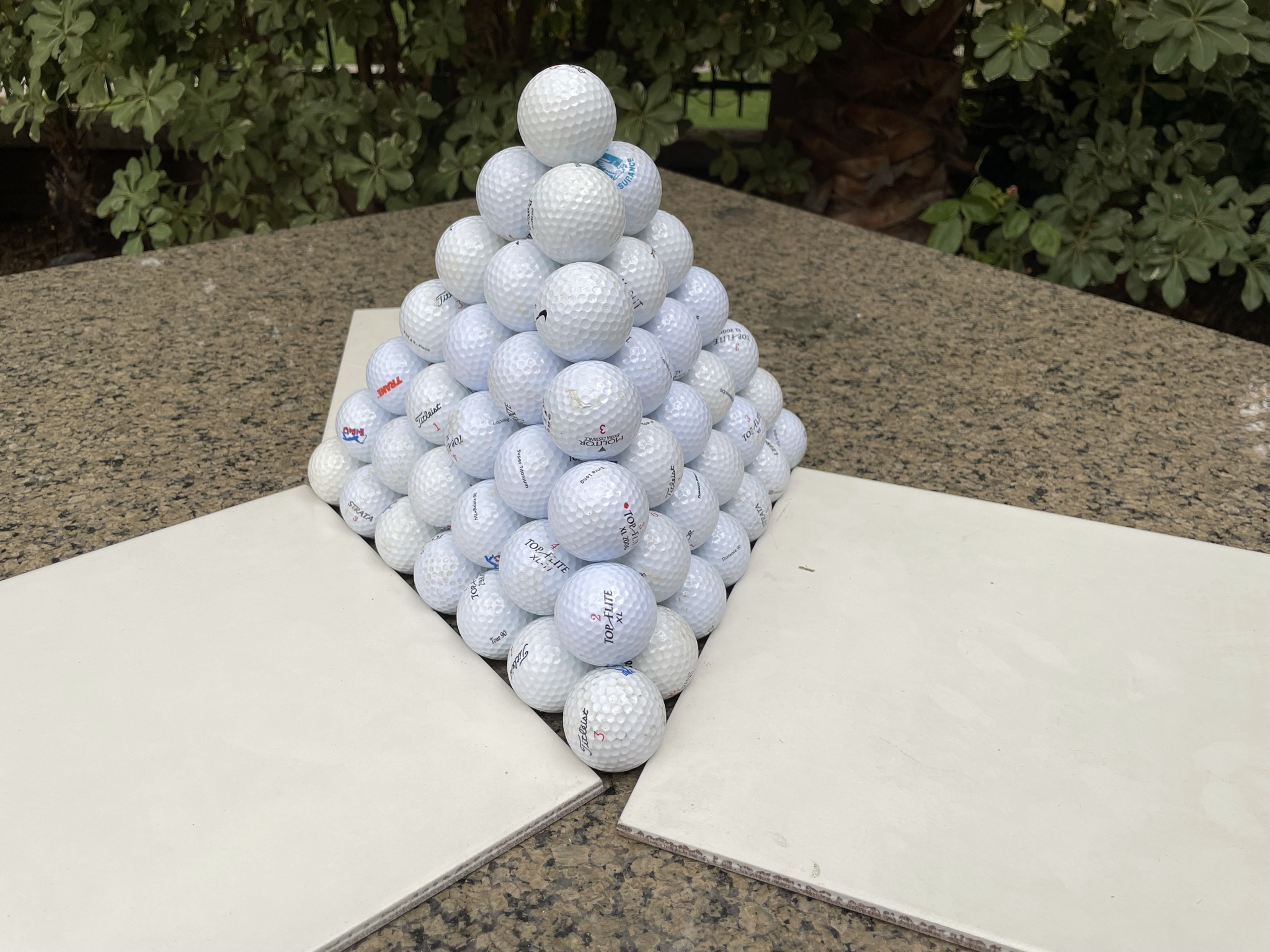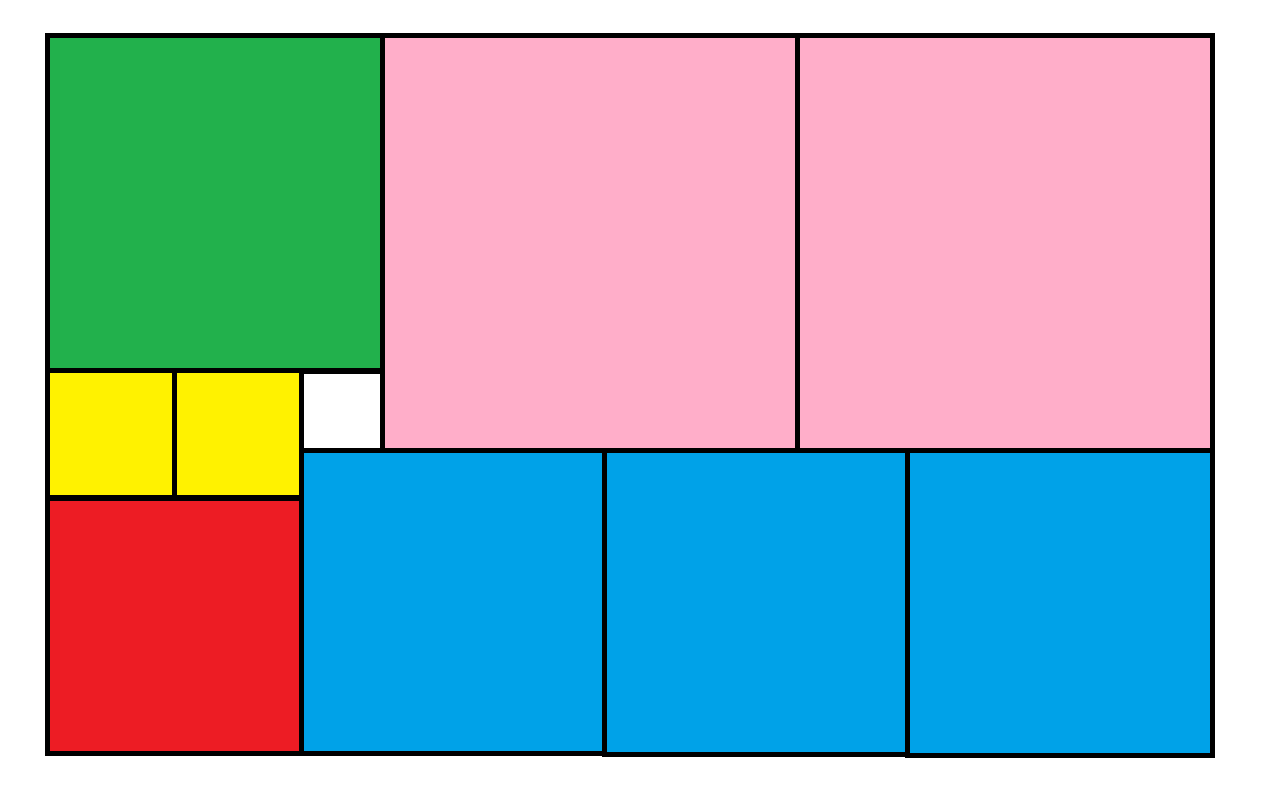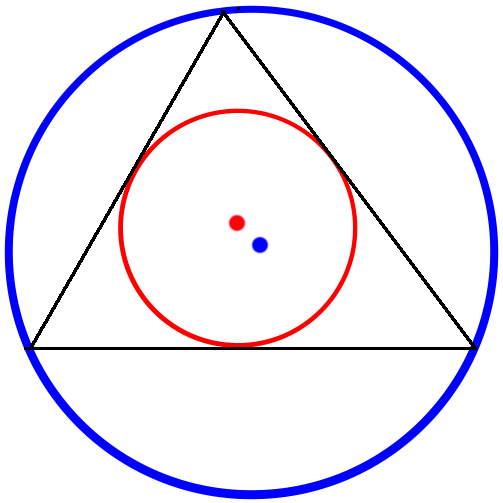## Poll

 I love math!17 votes (50%) Math is great.13 votes (38.23%) My religion is mathology.5 votes (14.7%) Women didn't speak to me until I was 30.2 votes (5.88%) Total eclipse reminder -- 04/08/202411 votes (32.35%) I steal cutlery from restaurants.3 votes (8.82%) I should just say what's on my mind.6 votes (17.64%) Who makes up these awful names for pandas?5 votes (14.7%) I like to touch my face.10 votes (29.41%) Pork chops and apple sauce.8 votes (23.52%)

34 members have voted

ThatDonGuyJoined: Jun 22, 2011
• Posts: 4962
August 31st, 2021 at 7:53:45 PM permalink
For efficiency calculation purposes, how is the "volume of the whole pyramid" measured - the volume of the circumscribing pyramid (I just had a 40-year-old flashback to an AMC exam problem I had in high school), or the volume of the pyramid whose vertices are the centers of the golf balls at each vertex?
WizardJoined: Oct 14, 2009
• Posts: 23444
September 1st, 2021 at 6:26:21 AM permalink
Quote: ThatDonGuy

For efficiency calculation purposes, how is the "volume of the whole pyramid" measured - the volume of the circumscribing pyramid (I just had a 40-year-old flashback to an AMC exam problem I had in high school), or the volume of the pyramid whose vertices are the centers of the golf balls at each vertex?

As the number of cannonballs approaches infinity, it shouldn't matter how exactly the volume is of the pyramid is measured.

However, if you must have an answer, let's keep it simple and say the length of a side of the base is the sum of the diameters of the cannonballs in a that side. For the height and slant height, use the same logic, keeping the angles in the pyramid correct.
It's not whether you win or lose; it's whether or not you had a good bet.
ssho88Joined: Oct 16, 2011
• Posts: 552
September 1st, 2021 at 7:23:10 AM permalink
Quote: Wizard

As the number of cannonballs approaches infinity, it shouldn't matter how exactly the volume is of the pyramid is measured.

However, if you must have an answer, let's keep it simple and say the length of a side of the base is the sum of the diameters of the cannonballs in a that side. For the height and slant height, use the same logic, keeping the angles in the pyramid correct.

r = radius of golf ball.

For square base, imagine a ball put on top of 4 balls and you join the center of five balls to form a square base pyramid, the vertical height of square base pyramid = 2^0.5 * r = 1.414r, and the slant distance = 2r.

For triangular base, imagine a ball put on top of 3 balls and you join the center of four balls to form a triangular base pyramid, the vertical height of triangular base pyramid = 2/3 * 6^0.5 * r = 1.633r, and the slant distance = 2r.

From there, we can calculate the total vertical height of N layers of balls, Am I missing something ?

ThatDonGuyJoined: Jun 22, 2011
• Posts: 4962
September 1st, 2021 at 7:47:19 AM permalink

Assume the "volume of the shape" is the volume of the tetrahedron/pyramid whose vertices are the centers of the cannonballs at the vertices.
Also assume each cannonball has radius 1.

If there are N levels:
The number of cannonballs in the tetrahedron is N (N^2 + 3 N + 2) / 6
The combined volume of the cannonballs is N (N^2 + 3 N + 2) / 6 * 4 PI / 3
= 2 PI (N^3 + 3 N^2 + 2 N) / 9

The tetrahedron is a regular tetrahedron of side length 2 (N - 1)
The volume is (2 (N - 1))^3 / (6 sqrt(2))
= 2 sqrt(2) / 3 * (N^3 - 3 N^2 + 3 N - 1)

The number of cannonballs in the pyramid is N (2 N^2 + 3 N + 1) / 6
The combined volume of the cannonballs is N (2 N^2 + 3 N + 1) / 6 * 4 PI / 3
= 2 PI (2 N^3 + 3 N^2 + N) / 9

The pyramid has sides of length 2 (N - 1)
The area of the base is 4 (N - 1)^2, and the height is (N - 1) sqrt(2),
The volume is 4 (N - 1)^2 * (N - 1) sqrt(2) / 3
= 4 sqrt(2) * (N^3 - 3 N^2 + 3 N - 1) / 3

The tetrahedron's efficiency is
(2 PI (N^3 + 3 N^2 + 2 N) / 9) / (2 sqrt(2) * (N^3 - 3 N^2 + 3 N - 1) / 3)
= PI / (3 sqrt(2)) * (N^3 + 3 N^2 + 2 N) / (N^3 - 3 N^2 + 3 N - 1)
As N approaches infinity, this approaches PI * sqrt(2) / 6

The pyramid's efficiency is
(2 PI (2 N^3 + 3 N^2 + N) / 9) / (4 sqrt(2) * (N^3 - 3 N^2 + 3 N - 1) / 3)
= PI / (3 sqrt(2)) * (N^3 + 3/2 N^2 + 1/2 N) / (N^3 - 3 N^2 + 3 N - 1)
As N approaches infinity, this also approaches PI * sqrt(2) / 6

The two have equal efficiency - specifically, PI sqrt(2) / 6

unJonJoined: Jul 1, 2018
• Posts: 2932
September 1st, 2021 at 7:56:32 AM permalink
Quote: Wizard

Which method of stacking cannonballs is the most efficient -- a pyramid with a square base or triangular?For full credit, I'd like to the degree of efficiency (meaning ratio of the volume of cannonballs to to the volume of the whole pyramid), both ways, as the number of cannonballs approaches infinity?

There will probably be an extra credit problem coming up, but one thing at a time.

Good problem. One of my calc professors in college solved the Kepler conjecture (ie, what�s the most efficient way to pack spheres). When I was in his class was before he solved it, but he was obsessed with it. Talked about it during multiple classes. He had reduced the problem to a differential equation but was unable to integrate it. A few years later he managed to find the integral and solved it.
The race is not always to the swift, nor the battle to the strong; but that is the way to bet.
WizardJoined: Oct 14, 2009
• Posts: 23444
September 1st, 2021 at 8:42:07 AM permalink
Quote: ThatDonGuy

Assume the "volume of the shape" is the volume of the tetrahedron/pyramid whose vertices are the centers of the cannonballs at the vertices.
Also assume each cannonball has radius 1.

If there are N levels:
The number of cannonballs in the tetrahedron is N (N^2 + 3 N + 2) / 6
The combined volume of the cannonballs is N (N^2 + 3 N + 2) / 6 * 4 PI / 3
= 2 PI (N^3 + 3 N^2 + 2 N) / 9

The tetrahedron is a regular tetrahedron of side length 2 (N - 1)
The volume is (2 (N - 1))^3 / (6 sqrt(2))
= 2 sqrt(2) / 3 * (N^3 - 3 N^2 + 3 N - 1)

The number of cannonballs in the pyramid is N (2 N^2 + 3 N + 1) / 6
The combined volume of the cannonballs is N (2 N^2 + 3 N + 1) / 6 * 4 PI / 3
= 2 PI (2 N^3 + 3 N^2 + N) / 9

The pyramid has sides of length 2 (N - 1)
The area of the base is 4 (N - 1)^2, and the height is (N - 1) sqrt(2),
The volume is 4 (N - 1)^2 * (N - 1) sqrt(2) / 3
= 4 sqrt(2) * (N^3 - 3 N^2 + 3 N - 1) / 3

The tetrahedron's efficiency is
(2 PI (N^3 + 3 N^2 + 2 N) / 9) / (2 sqrt(2) * (N^3 - 3 N^2 + 3 N - 1) / 3)
= PI / (3 sqrt(2)) * (N^3 + 3 N^2 + 2 N) / (N^3 - 3 N^2 + 3 N - 1)
As N approaches infinity, this approaches PI * sqrt(2) / 6

The pyramid's efficiency is
(2 PI (2 N^3 + 3 N^2 + N) / 9) / (4 sqrt(2) * (N^3 - 3 N^2 + 3 N - 1) / 3)
= PI / (3 sqrt(2)) * (N^3 + 3/2 N^2 + 1/2 N) / (N^3 - 3 N^2 + 3 N - 1)
As N approaches infinity, this also approaches PI * sqrt(2) / 6

The two have equal efficiency - specifically, PI sqrt(2) / 6

I agree!
It's not whether you win or lose; it's whether or not you had a good bet.
WizardJoined: Oct 14, 2009
• Posts: 23444
September 3rd, 2021 at 3:15:52 PM permalinkThe above figure contains 10 squares inscribed in a rectangle. The white square has side length of 1. What is the area of the entire rectangle?

It's not whether you win or lose; it's whether or not you had a good bet.
charliepatrickJoined: Jun 17, 2011
• Posts: 2444
September 3rd, 2021 at 3:44:03 PM permalink
Quote: Wizard

...The above figure contains 10 squares inscribed in a rectangle. The white square has side length of 1. What is the area of the entire rectangle?...

Simultaneous equations gets it...
Let Yellow = x
Green = 2x+1
Pink = 2x+2
Red = 2x
Blue = 3x-1

Then look at the widths 2 Pink + Green (6x+5) or 3 Blue + Red (11x-3) giving x=8/5
So width = 14 3/5 and Height = (Pink+Blue) 5x+1 = 9
So area = 131.4.
WizardJoined: Oct 14, 2009
• Posts: 23444
Thanks for this post from:September 3rd, 2021 at 8:49:24 PM permalink
Quote: charliepatrick

Simultaneous equations gets it...

Let Yellow = x
Green = 2x+1
Pink = 2x+2
Red = 2x
Blue = 3x-1

Then look at the widths 2 Pink + Green (6x+5) or 3 Blue + Red (11x-3) giving x=8/5
So width = 14 3/5 and Height = (Pink+Blue) 5x+1 = 9
So area = 131.4.

I agree!
It's not whether you win or lose; it's whether or not you had a good bet.
ThatDonGuyJoined: Jun 22, 2011
• Posts: 4962
September 4th, 2021 at 9:06:18 AM permalink
Here's a problem that's as easy or as hard as you want it to beTriangle ABC has side lengths of 13, 14, and 15.
X is the center of the inscribed circle - that is, the circle that is tangent to all three sides. (In the image, it is in red.)
Y is the center of the circumscribed circle - that is, the circle that goes through A, B, and C. (In the image, it is in blue.)
What is the distance from X to Y?

If you want to make it easier, here are three hints:

The radius of the inscribed circle is 4

The radius of the circumscribed circle is 65/8

If AB has length 13 and BC has length 15, the distance from B to the point where the inscribed circle touches BC is 7.